The ‘gggrid’ package provides two functions: grid_panel() and grid_group(). Both functions add ‘grid’ output to a ‘ggplot2’ plot.

The grid_panel() function is called once for each panel in a (facetted) ‘ggplot2’ plot and the grid_group() function is called once for each group in a ‘ggplot2’ plot.

The first argument to both functions can be a ‘grid’ grob. For example, the following code defines a text grob that will be drawn 5mm in from the top-right corner of wherever it is drawn.

library(grid)
label <- textGrob("Label",
x=unit(1, "npc") - unit(5, "mm"),
y=unit(1, "npc") - unit(5, "mm"),
just=c("right", "top"))

The following code calls grid_panel() to add that text label to a ‘ggplot2’ plot.

library(gggrid)
## Loading required package: ggplot2
ggplot(mtcars) +
geom_point(aes(disp, mpg)) +
grid_panel(label)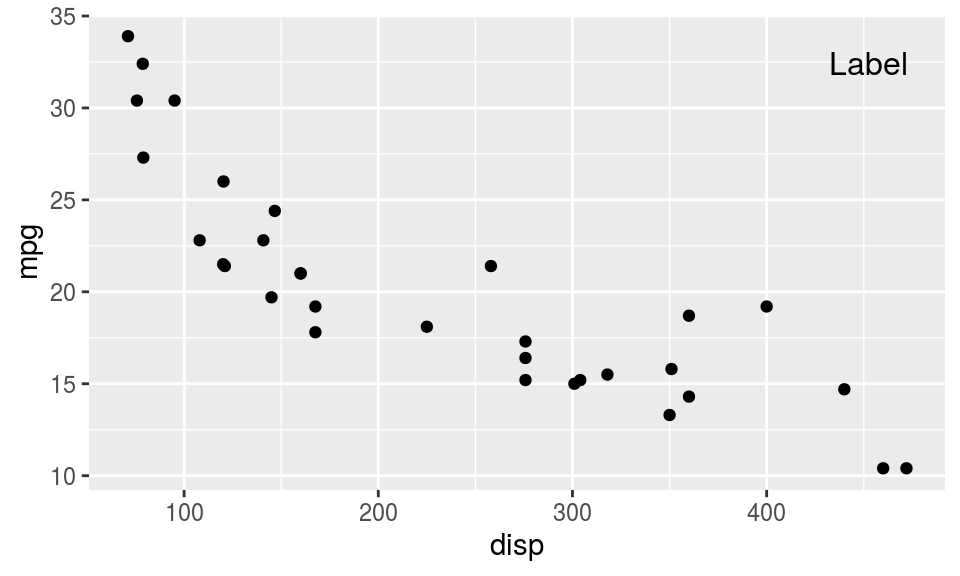The first argument to both functions can also be a function that generates a grob. The function must accept two arguments, data and coords, which contain the data values (and transformed data values) for the ‘ggplot2’ panel that is being drawn. For example, the following code adds a “rug” of short lines showing the distribution of the y-values in the plot.

rug <- function(data, coords) {
segmentsGrob(unit(1, "npc"),
coords$y, unit(1, "npc") - unit(2, "mm"), coords$y,
gp=gpar(lwd=2, col=rgb(0,0,0,.5)))
}
ggplot(mtcars, aes(x=disp, y=mpg)) +
geom_point() +
grid_panel(rug)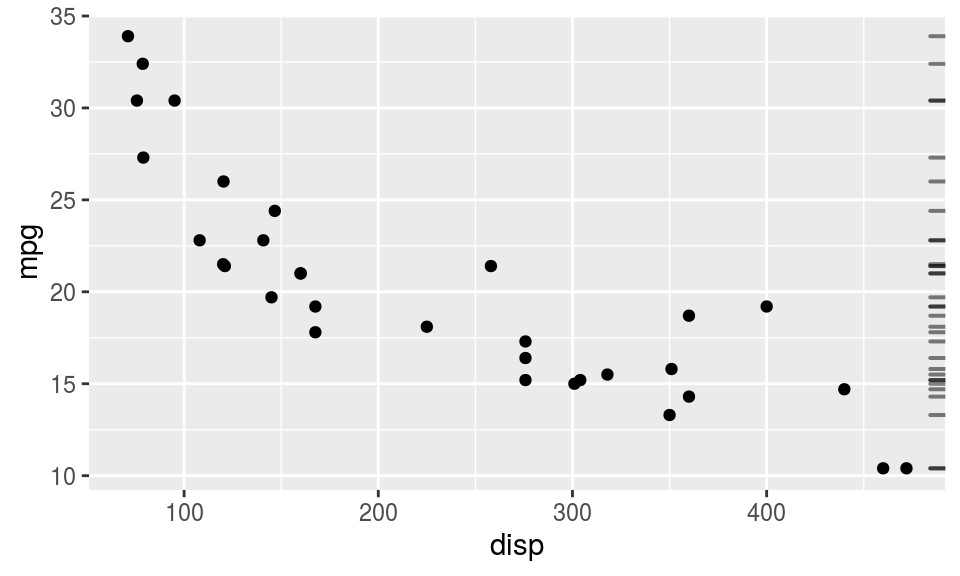The following code adds facetting to demonstrate that grid_panel() is called once for panel.

ggplot(mtcars, aes(x=disp, y=mpg)) +
geom_point() +
grid_panel(rug) +
facet_wrap("am")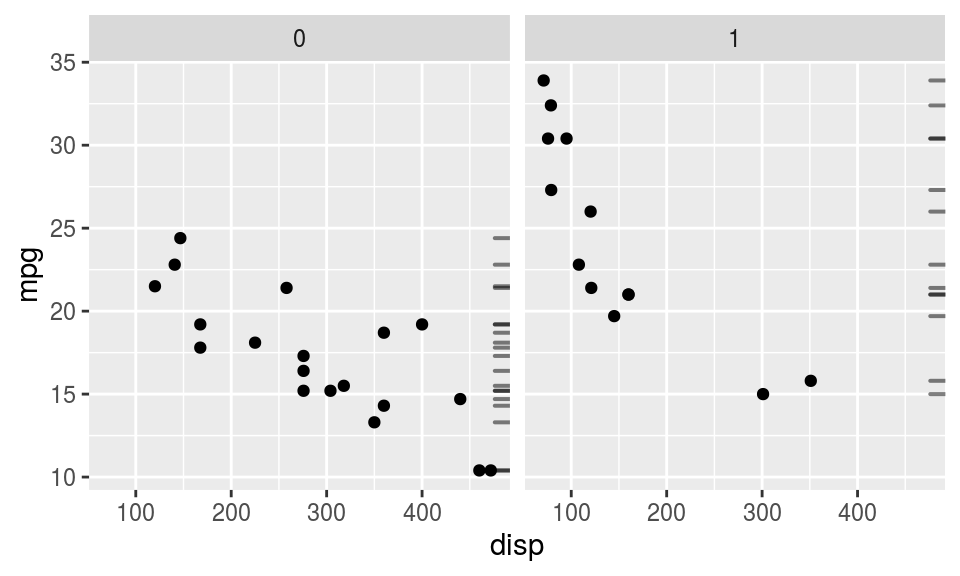The following code adds grouping to demonstrate that grid_group() is called once for each group.

rugGroup <- function(data, coords) {
segmentsGrob(unit(1, "npc"),
coords$y, unit(1, "npc") - unit(2, "mm"), coords$y,
gp=gpar(lwd=2, col=adjustcolor(data$colour, alpha=.5))) } ggplot(mtcars, aes(x=disp, y=mpg, colour=as.factor(am))) + geom_point() + grid_group(rugGroup)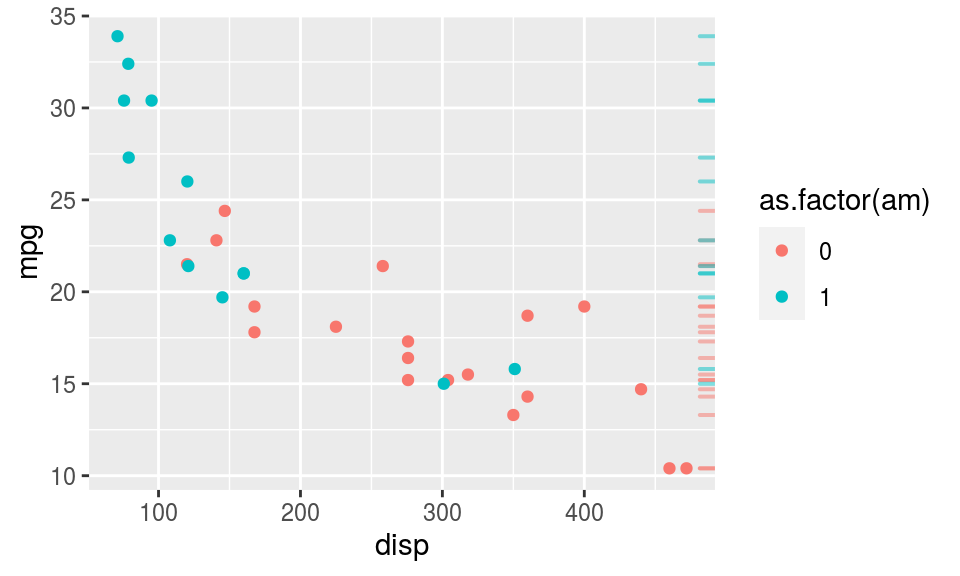The following code demonstrates that ‘gggrid’ is one way to access ‘grid’-based drawing that is not (yet) available via ‘ggplot2’-based geom_*() functions. In this case, we make use of variable-width lines from the ‘vwline’ package. minard <- read.table("minard-troops.txt", header=TRUE) library(vwline) path <- function(data, coords) { vwlineGrob(coords$x, coords$y, w=unit(coords$size, "in"),
gp=gpar(col=coords$colour, fill=adjustcolor(coords$colour, alpha=.5)))
}
ggplot(minard,
aes(x=long, y=lat, size=survivors, colour=direction,
group=interaction(group, direction))) +
scale_size(range=c(.01, .5)) +
coord_fixed(2, clip="off") +
grid_group(path)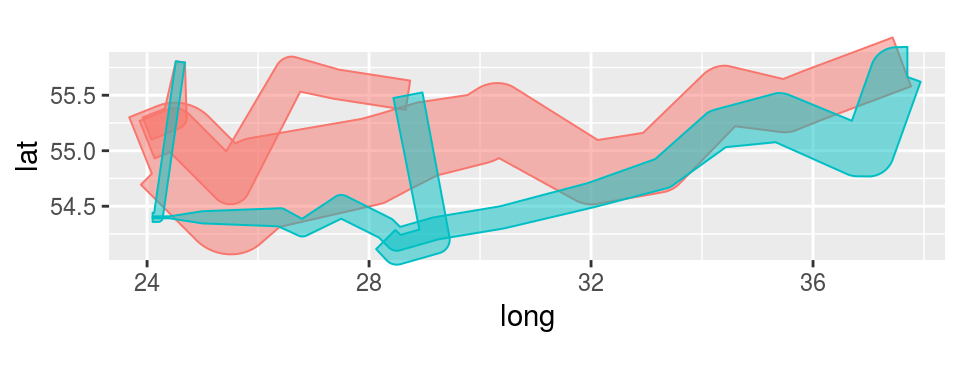The above plot has no legend, but it is possible to add one with a little more work. There are three important steps:

1. Set show.legend=TRUE
2. Set key_glyph to be a function that produces a grob (to draw an item in the legend)
3. Set an explicit mapping in the grid_group() call (rather than just having grid_group() inherit a mapping).

The following code demonstrates these steps, including defining a path_key() function that draws a variable-width line in the legend, to show the relationship between colour and direction.

We have excluded the size legend in this case (with guide="none") to keep the path_key() function relatively simple.

path_key <- function(data, ...) {
vwlineGrob(0:1, c(.5, .5), w=unit(c(.1, .2), "in"),
gp=gpar(col=data$colour, fill=adjustcolor(data$colour, alpha=.5)))
}
ggplot(minard) +
scale_size(range=c(.01, .5), guide="none") +
coord_fixed(2, clip="off") +
grid_group(path,
aes(x=long, y=lat, size=survivors, colour=direction,
group=interaction(group, direction)),
show.legend=TRUE,
key_glyph=path_key)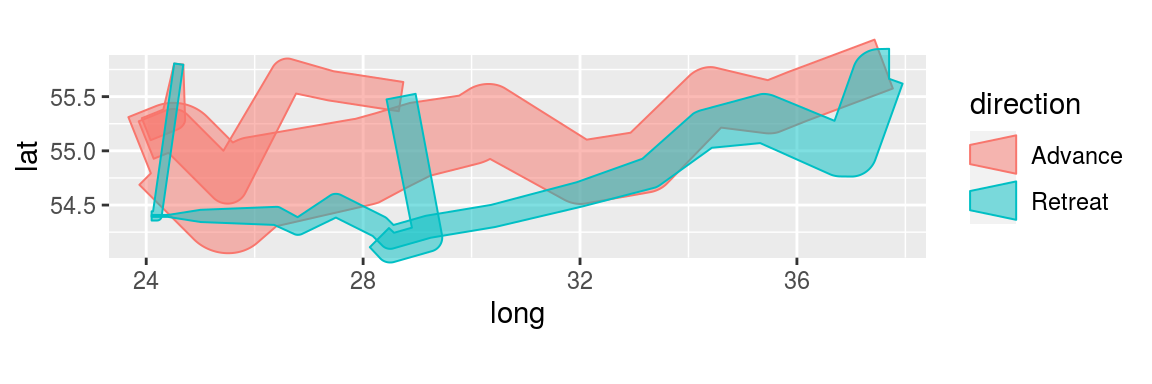The Minard data used in the example above comes from the supplementary materials that were published with Wickham (2010).